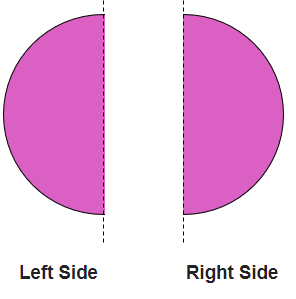Q.1) Find the Perimeter of the given triangle: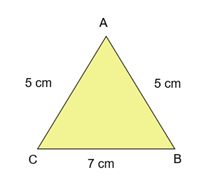a) 17 cm
b) 14 cm
c) 15 cm

Q.2) Find the Perimeter of the given Rectangle: –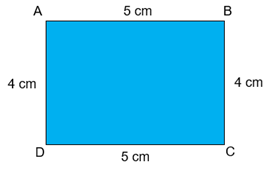a) 16 cm
b) 14 cm
c) 18 cm

Q.3) A rectangle has _____ sides.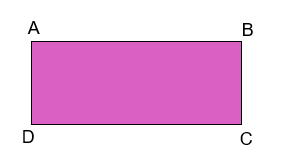a) 5
b) 3
c) 4

Q.4) How many vertices does the given figure have?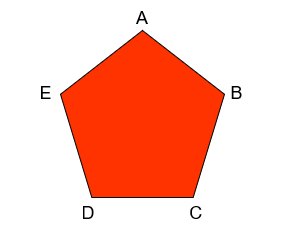a) 6
b) 4
c) 5

Q.5) Identify the given figure ?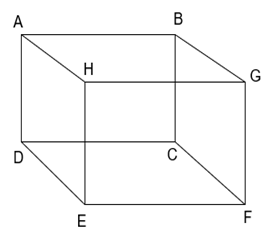a) cube
b) cuboid
c) sphere

Q.6) How many edges does the given figure have?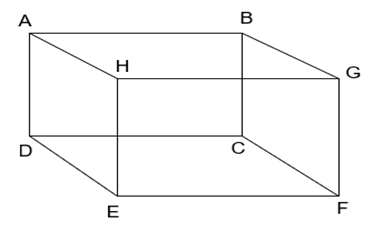a) 8
b) 6
c) 12

Q.7) _______ has no end point.
a) Line segment
b) Line
c) Ray

Q.8) _______ has two end points.
a) Line segment
b) Line
c) Ray

Q.9) Is the given figure, symmetrical about the dotted line?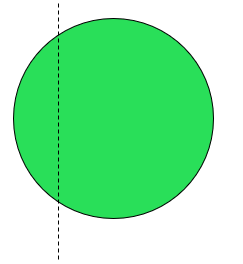a) Yes
b) No

Q.10) Is the given figure is symmetrical about the dotted line?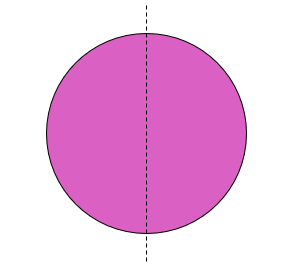a) Yes
b) No

### Geometry Worksheets Grade 3 Explanation

Q.1) Explanation – Geometry Worksheets Grade 3

Perimeter is the sum of all the sides of a closed figure.
Perimeter = Sum of all the sides
Perimeter of the given Triangle = AB + BC + CA
Perimeter of the given Triangle = 5 cm + 7 cm + 5 cm
Perimeter of the given Triangle = 17 cm

Correct Answer – a) 17 cm

Q.2) Explanation – Geometry Worksheets Grade 3

Perimeter is the sum of all the sides of a closed figure.
Perimeter = Sum of all the sides
Perimeter of given Rectangle = AB + BC + CD + DA
Perimeter of given Rectangle = 5 cm + 4 cm + 5 cm + 4 cm
Perimeter of given Rectangle = 18 cm

Correct Answer – c) 18 cm

Q.3) Explanation – Geometry Worksheets Grade 3

A rectangle has 4 sides.
In the above figure, the 4 sides are:
AB, BC, CD and DA

Q.4) Explanation –

A vertex is a corner, where two edges meet.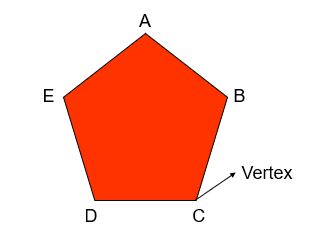In the given figure
A is a vertex, as edge of line AE and AB meet at point A.
B is a vertex, as edge of line AB and BC meet at point B.
C is a vertex, as edge of line BC and CD meet at point C.
D is a vertex, as edge of line CD and DE meet at point D.
E is a vertex, as edge of line DE and EA meet at point E.
Hence, the given figure has 5 vertices.

Q.5) Explanation – Geometry Worksheets Grade 3

A cube has 6 faces, 12 edges and 8 vertices.
All six faces of a cube are square.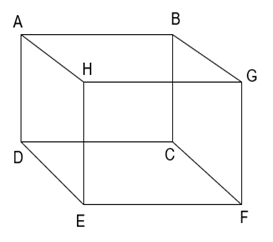In the given figure, there are 6 faces, 12 edges and 8 vertices.
All six faces of the given figure are square.
Hence, the given figure is cube.

Q.6) Explanation –

Edge is the side where two adjacent faces meet.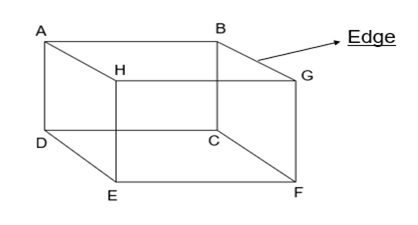There are 12 edges in the given figure.
In the given figure 12 edges are:
AB, BC, CD, DA, AH, BG, CF, DE, GH, HE, EF and FG

Q.7) Explanation –

Line has no end point. It can be extended infinitely from both sides.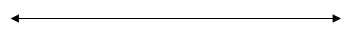Q.8) Explanation – Geometry Worksheets Grade 3

Line segment has two end points.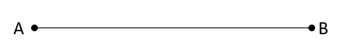In the given diagram, Line segment AB has two end points A and B.

Correct Answer – a) Line segment

Q.9) Explanation –

A figure is said to be symmetrical about the dotted line, if on folding, one part will fit exactly over the other part.

If we separate the given image on the dotted line, it would be like this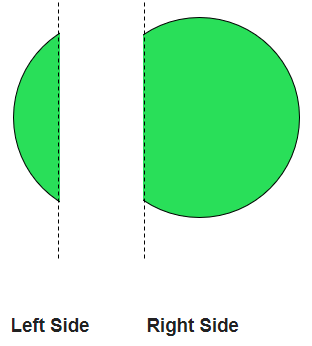Since the image on the left side of the dotted line is different in shape from the image on the right side of the dotted line, on folding, left side image would not exactly over the right side image. Hence, we can say that the given figure is not symmetrical about dotted line.